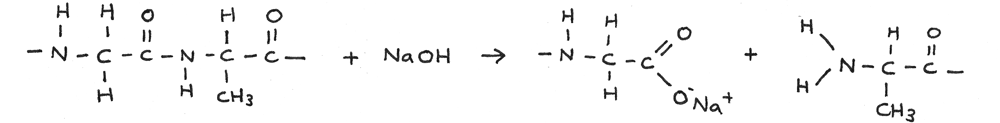# Amines And Amino Acids

## Amines

Amines are derivatives of ammonia whereby one or more hydrogen atoms have been replaced by alkyl groups. Amines can be primary, secondary or tertiary depending on how many hydrogen atoms have been replaced.

• Primary amines: One alkyl group is attached to the nitrogen atom.
• Secondary amines: Two alkyl groups are attached to the nitrogen atom.
• Tertiary amines: All three hydrogen atoms are replaced by alkyl groups.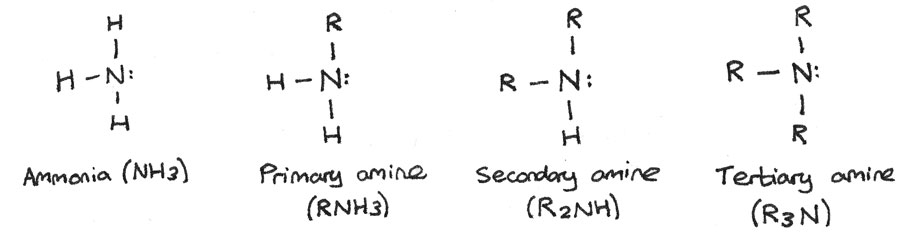### Naming Amines

The naming of amines is based around the length of the longest alkyl chain connected to the nitrogen atom. Below are some examples of amines:

#### Primary Amine

$$CH_{3}CH_{2}CH_{2}CH_{2}NH_{2}$$
This is butylamine, a primary amine. The -butyl part comes from the four carbon alkyl group while the -amine part is due to the secondary amine group, $NH_{2}$.

#### Secondary Amine

$$CH_{3}(CH_{3}CH_{2}CH_{2})NH$$
This is N-methylpropylamine. The -propyl part comes from the longer three carbon chain while the -methyl part is from the other $CH_{3}$ group. The preceding N indicates that the methyl group is attached to the nitrogen atom.

#### Tertiary Amine

$$(CH_{3 }CH_{2})_{2}CH_{3}CH_{2}CH_{2}N$$
This is N,N-diethylpropylamine. The -propyl part comes from the longer three carbon chain while the diethyl is from the two ethyl groups connected to the nitrogen atom.

### Amine as Weak Base

Amines are weak bases. This is because a lone pair of electrons on the nitrogen atom can accept a proton ($H^{+}$). When the amine accepts the proton, a dative covalent bond is formed between the amine and the proton.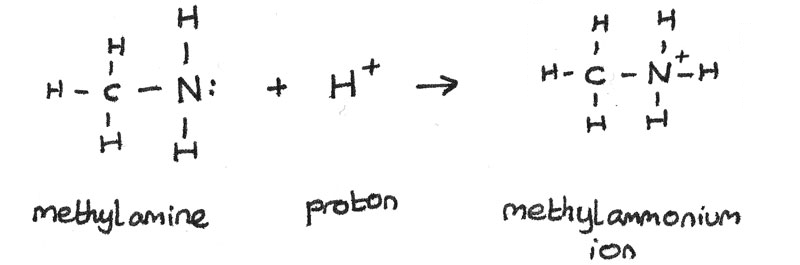Amines can react with acids to produce alkylammonium salts. For example, ethylamine can react with hydrochloric acid to form ethylammonium chloride.
$$CH_{3}CH_{2}NH_{2(aq)} + HCl_{(aq)} \rightarrow CH_{3}CH_{2}NH_{3}^{+}Cl^{-}_{(aq)}$$

### Preparation of Aliphatic Amines

Aliphatic amines can be prepared by warming halogenoalkanes with an excess of ammonia, using ethanol as a solvent.

The formation of propylamine through the reaction between 1-chloropropane and ammonia is shown below:
$$CH_{3}CH_{2}CH_{2}Cl + NH_{3} \rightarrow CH_{3}CH_{2}CH_{2}NH_{2} + HCl$$

As the propylamine also has a lone pair of electrons on the nitrogen atom, further nucleophilic substitution can occur, forming a secondary amine. The ammonia acts as a nucleophile and attacks the $\delta +$ carbon atom in the carbon-halogen bond.

$$CH_{3}CH_{2}CH_{2}Cl + CH_{3}CH_{2}CH_{2}NH_{2} \rightarrow (CH_{3}CH_{2}CH_{2})_{2}NH + HCl$$

To minimise the further substitution, a very large excess of ammonia can be used.

### Preparation of Aromatic Amines

To prepare an aromatic amine from benzene, the benzene ring must first be nitrated to form nitrobenzene. Nitrobenzene is used in the synthesis of dyes and in pharmaceutical products. This is done by reacting benzene with concentrated nitric acid. A catalyst of concentrated sulphuric acid is used at a temperature of 50°C.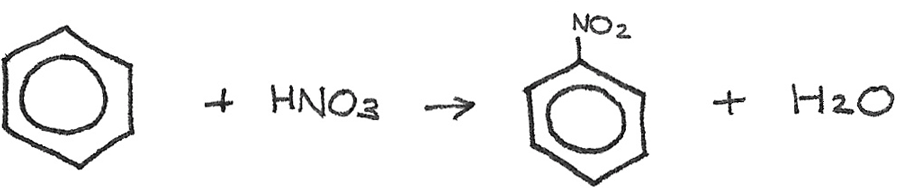Next the nitrobenzene can be reduced to phenylamine by reacting it with a mixture of tin and concentrated hydrochloric acid, heated under reflux. After the reaction, the excess hydrochloric acid should be neutralised. The tin and hydrochloric acid act as reducing agents, shown by $[H]$ in the reaction.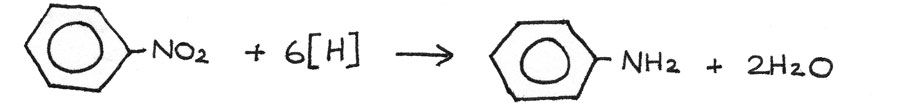## Synthesis of Dyes

Aromatic amines can be used in the production of industrial dyes. It is performed in two stages: diazotisation and coupling.

### Diazotisation

In the diazotisation process, the phenylamine is converted into a diazonium salt. This is done by reacting phenylamine with nitrous acid below 10°C. The nitrous acid ($HNO_{2}$) is generated in the reaction mixture by reacting sodium nitrite ($NaNO_{2}$) with hydrochloric acid ($HCl$).

$$NaNO_{2} + HCl \rightarrow HNO_{2} + NaCl$$

The nitrous acid reacts with an aromatic amine to form a diazonium salt. In this case, benzenediazonium chloride is produced: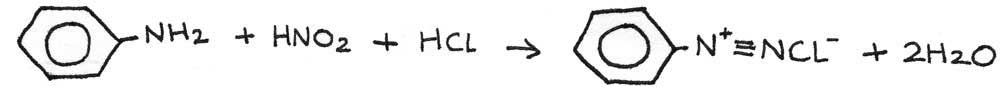### Coupling

The diazonium salt can then be reacted with another aromatic compound in order to produce an azo compound. An azo compound is a compound containing the azo functional group, $-N=N-$.

In the reaction below, the benzenediazonium chloride is reacted with phenol and sodium hydroxide. The diazonium salt acts as an electrophile. This reaction is performed under alkaline conditions.## Amino Acids

Amino acids are compounds which contain both an amine group ($-NH_{2}$) and a carboxylic acid group ($-COOH$). They are important in the human body as they are building blocks for proteins and peptides. They also act as enzymes and antibodies.

### $\alpha$-amino acids

$\alpha$-amino acids have a basic amine group and an acidic carboxyl group bonded to the same carbon atom. The general formula is $RCH(HN_{2})COOH$. The body has 20 amino acids that can be arranged to form proteins. The simplest $\alpha$-amino acid is glycine which has a hydrogen atom as the $-R$ group.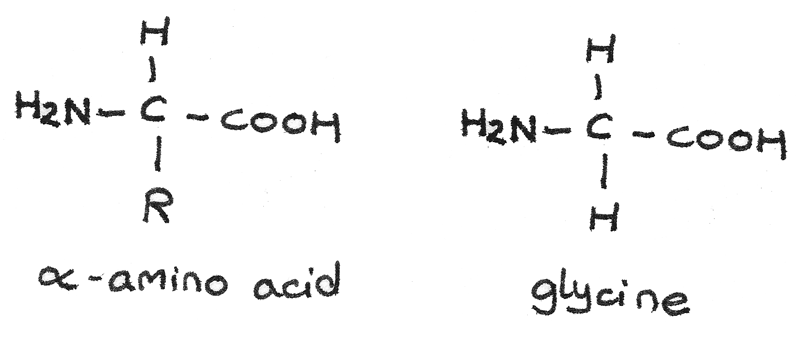Other examples of $\alpha$-amino acids include alanine (2-aminopropanoic acid) and glutamic acid (2-aminopentanedioic acid).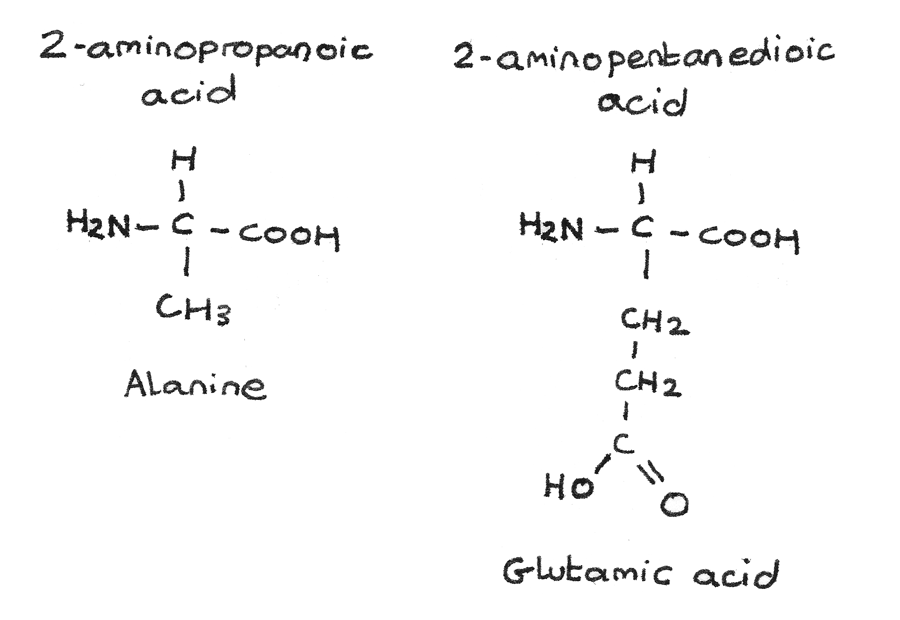### Zwitterions

The acidic carboxyl group and the basic amine group in an amino acid can interact with each other to form an internal salt known as a zwitterion. In this process:

• A proton is transferred from the acid carboxyl group to the basic amine group.
• The zwitterion has no overall net charge as the positive and negative charges cancel each other out.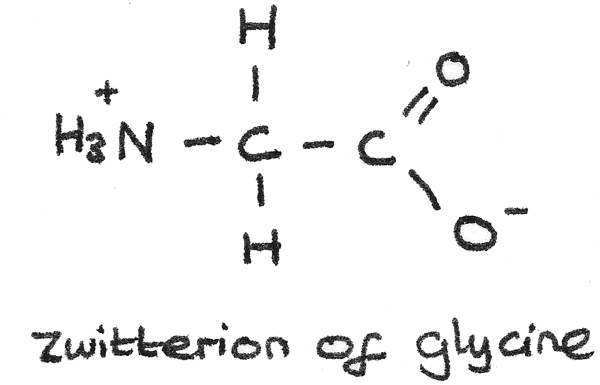### Isoelectric Point

The isoelectric point is the pH at which there is no net electrical charge. At this pH, a zwitterion is formed. Different amino acids have different isoelectric points. A higher isoelectric point indicates the $-R$ group may contain $-NH_{2}$ while a lower isoelectric point could show the presence of a $-COOH$ group.

### Acid and Base Properties

Amino acids are amphoteric, meaning they can react as an acid or a base.

• At a pH more acidic than the isoelectric point, the amino acid behaves as a base and accepts a proton from the acid. This results in a positively charged ion.
$$H_{3}^{+}NCH_{2}COO^{-} \rightarrow H^{+}_{3}NCH_{2}COOH$$

• At a pH less acidic than the isoelectric point, the amino acid behaves as an acid and donates a proton to the base. This results in a negatively charged ion.
$$H_{3}^{+}NCH_{2}COO^{-} \rightarrow H_{2}NCH_{2}COO^{-}$$

## Peptides

A peptide is a compound containing two or more amino acids joined by a peptide bond.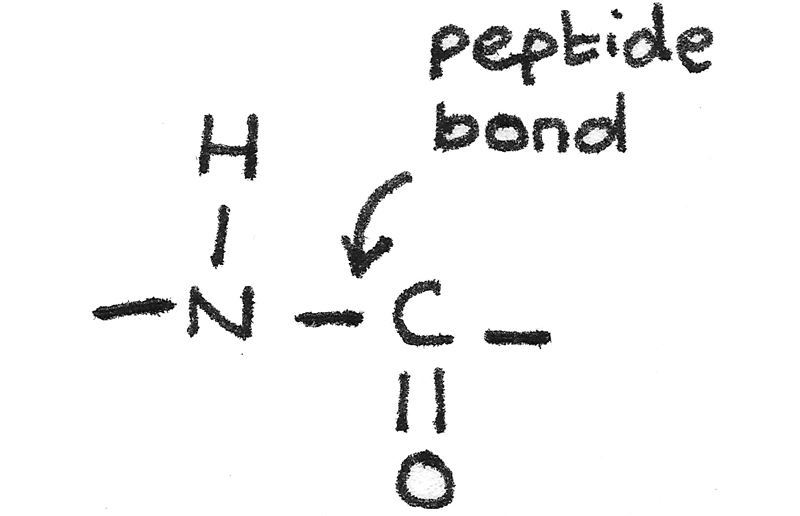When two amino acids join together, a dipeptide is formed through the elimination of water in a condensation reaction.It is also possible to form a dipeptide by reacting two of the same amino acids together. The amine group on one of the molecules reacts with the carboxyl group on the other.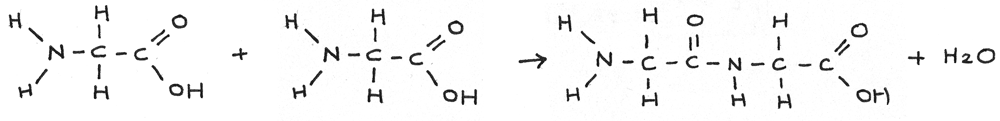## Polypeptides

A polypeptide is a long chain of amino acids joined together with peptide bonds. A polypeptide can be formed through a series of condensation reactions of amino acids. A dipeptide is a molecule formed from two amino acids joined by a single peptide bond.

### Acid Hydrolysis

Polypeptides can be hydrolysed using aqueous acid. In this reaction the peptide bond is broken forming two positively charged amino acids. This reaction is traditionally carried out by heating under reflux with $6~mol~dm^{-3}$ hydrochloric acid for 24 hours.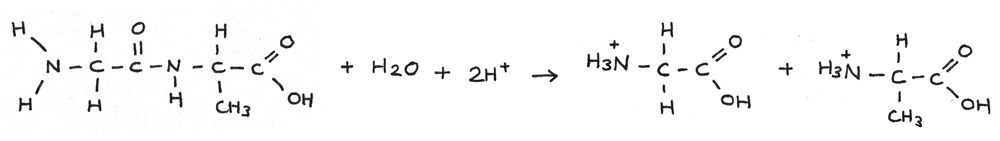### Alkaline Hydrolysis

Polypeptides can be broken down through alkaline hydrolysis. A solution of sodium hydroxide can be used just above 100° C. In this reaction the polypeptide is broken down into salts.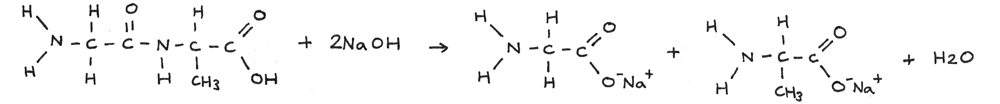In the reaction below a long amino acid chain is broken through alkaline hydrolysis.